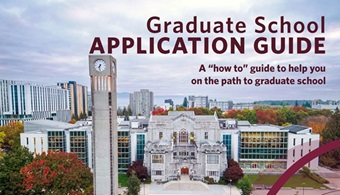# Yaniv Plan

Associate Professor

#### Affiliations to Research Centres, Institutes & Clusters

##### Doctoral Student Supervision (Jan 2008 - April 2022)
On LASSO parameter sensitivity (2021)

Compressed sensing (CS) is a paradigm in which a structured high-dimensional signal may be recovered from random, under-determined, corrupted linear measurements. LASSO programs are effective for solving CS problems due to their proven ability to leverage underlying signal structure. Three popular LASSO programs are equivalent in a sense and sometimes used interchangeably. Tuned by a governing parameter, each admits an optimal parameter choice yielding minimax order-optimal error. CS is well-studied, though theory for LASSO programs typically concerns this optimally tuned setting. However, the optimal parameter value for a LASSO program depends on properties of the data and is typically unknown in practical settings. Performance in empirical problems thus hinges on a program's parameter sensitivity: it is desirable that small variation about the optimal parameter choice begets small variation about the optimal risk.We examine the risk of three LASSO programs as a function of their governing parameters and further demonstrate that their parameter sensitivity can differ for the same data, thereby informing the selection of a LASSO program in practice. We prove a gauge-constrained program admits asymptotic cusp-like behaviour of its risk in the limiting low-noise regime; a residual-constrained program has asymptotically suboptimal risk for very sparse vectors (i.e., for any fixed parameter choice, the ratio of the risk to the optimally achievable risk grows unbounded). These results contrast observations about an unconstrained program with sufficiently large parameter. Our theory is supported with extensive numerical simulations, demonstrating the parameter sensitivity phenomenon for even modest dimensional parameters.We first analyze these risks for proximal denoising (PD), in which one directly observes signal plus noise (i.e., the measurement matrix is identity). There, we further reveal a data regime in which the unconstrained PD risk can be asymptotically suboptimal. We also show how our theory extends to analyze generalized LASSO programs for generalized CS. Finally, we extend a keystone of our theoretical analysis, the projection lemma. We generalize the result to an arbitrary Hilbert space and extend it from scaled projections to proximal mappings of a dilated gauge. We discuss applications and possible directions for these results.

View record

Near-optimal sample complexity for noisy or 1-bit tensor completion (2018)

Tensor completion is the problem of recovering a low-rank tensor from a partial subset of its entries. Assuming a rank-r, order-d tensor in ℝ^{NxNx...N}, the best sampling complexity achieved is O(rN^{d/2}) which can be obtained by a tensor nuclear-norm minimization problem. This bound is significantly larger than O(rdN), the number of free variables in a rank-r tensor. In this thesis, we prove that when r=O(1), we can achieve optimal sample complexity by constraining either one of two proxies for tensor rank, the convex M-norm or the non-convex max-qnorm. The max-qnorm is the generalization of matrix max-norm to tensors which is non-convex. The M-norm, on the other hand, is a convex atomic norm whose atoms are rank-1 sign tensors. We prove that both max-qnorm and M-norm of a bounded rank-r tensor are bounded by quantities that are independent of N. We also prove that the unit balls of both these norms have small Rademacher complexity.We analyze max-qnorm and M-norm constrained least squares minimization problems for tensor completion, proving that when r=O(1), m=O(Nd) measurements are sufficient for efficient estimation of the original tensor. We also use an information theoretic technique to prove that the dependence on N is optimal. Moreover, we design an alternating method for approximating the solution of max-qnorm tensor completion and do a thorough investigation of its performance on synthetic and real-world data.We also generalize the 1-bit matrix completion problem to higher-order tensors. We prove that when r=O(1) a bounded rank-r, order-d tensor T in ℝ^N x ℝ^N x ... x ℝ^N can be estimated efficiently by only m=O(Nd) binary measurements by regularizing either its max-qnorm or its M-norm. We prove that the sample complexity of recovering a low-rank tensor from 1-bit measurements of a subset of its entries is the same as recovering it from unquantized measurements. Moreover, we show the advantage of using 1-bit tensor completion over matricization both theoretically and numerically. Specifically, we show how the 1-bit measurement model can be used for context-aware recommender systems.

View record

#### Membership Status

Member of G+PS
View explanation of statuses

#### Department(s)

If this is your researcher profile you can log in to the Faculty & Staff portal to update your details and provide recruitment preferences.